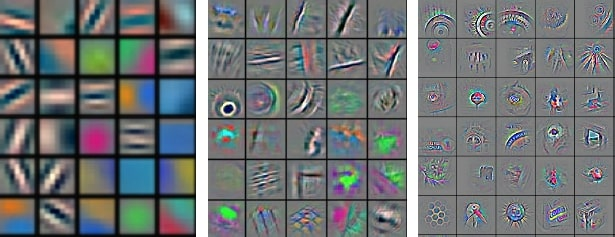### CNN with filter (Q24)

Hello,

In Q24 of the exam, what do they mean by "the data is laid out in a 1D fashion"?
Do we have to consider a filter taking M elements of the K nodes? Or does that mean that we output in 1D?
I do not understand how we pass from K^2 to M parameters per layer.

Thanks for the help,what do they mean by "the data is laid out in a 1D fashion"?
Do we have to consider a filter taking M elements of the K nodes? Or does that mean that we output in 1D?

"the data is laid out in a 1D fashion" = we flatten the input, e.g. a color image tensor (3D) and black-white image (2D) all get squeezed into a 1D vector. After the first hidden layer, all subsequent layers see a vector of $$K$$ by 1 features.

I do not understand how we pass from K^2 to M parameters per layer.

If the previous layer has $$K$$ nodes (neurons), and the next layer has $$K$$ neurons, with fully connected layers, there will be $$K^2$$ connections (=parameters) per layer.

Now, doing a convolution over the $$K$$ nodes (features) per layer, using a filter of size $$M$$, will take $$O(KM)$$ computations (assuming $$M << K$$). However, since we only use 1 filter per hidden layer, and every node uses that same filter (= weight sharing), we only have $$M$$ parameters per layer.

However, since we only use 1 filter per hidden layer, and every node uses that same filter (= weight sharing), we only have $$M$$ parameters per layer.

Where is this written in the question? I mean, is it indicated that we should use only one filter per layer? Because when I read it, I though that everyone of the K nodes at each layer would connect to M nodes of the subsequent layer, therefore I put KM.

Where is this written in the question? I mean, is it indicated that we should use only one filter per layer?

The key difference between a convolutional (Conv) and fully connected (FC) layer is: in Conv all neurons of the same layer share the $$M_i$$ weights (for the same filter $$i$$). Generally, in a ConvNet it is possible to have multiple filters per layer (leading to multiple output "channels"), but in this case the question states "the filter", thus referring to a single filter.

In CNN, what is the role of the number of nodes per layer?
My understanding so far is that the input nodes are the pixels and the hidden nodes the output of the convolutions
Do we decide the number of nodes or are they automatically decided by the kernel size, stride and padding?

In CNN, what is the role of the number of nodes per layer?
Do we decide the number of nodes or are they automatically decided by the kernel size, stride and padding?

Correct, the number of nodes in a convolutional layer is determined by the input feature size, kernel (=filter) size, padding, stride, ... and the convolutional operation applied to it.

By allowing for multiple filters (with different weights), you can detect significantly different features. E.g. in images: different colors, black-white edges, white-black edges, different shapes, ...

E.g. in this figure every square is a different filter (set of weights):Image creds: https://arxiv.org/abs/1311.2901

My understanding so far is that the input nodes are the pixels and the hidden nodes the output of the convolutions

Almost completely correct. Note that convolutional layers can also work on features from hidden layers, which do not directly have a pixel interpretation.

To get a visual idea of what is happening inside a CNN, check this demo (start by drawing a number): https://www.cs.ryerson.ca/~aharley/vis/conv/flat.html

Page 1 of 1